Multiplying

When multiplying a monomial (or one term expression) by a binomial (or two-term expression), the Distributive Property will be used to multiply the two functions.

Example Given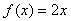and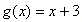, find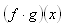.

Step 1. Replace f(x) and g(x) with their equivalent expressions.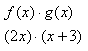Step 2. Use the Distributive Property to multiply and simplify.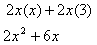For a review of the Distributive Property, select the following link:

Listen